## R语言基于poLCA包进行潜类别分析

news/2023/6/6 3:36:51

``````library(poLCA)
``````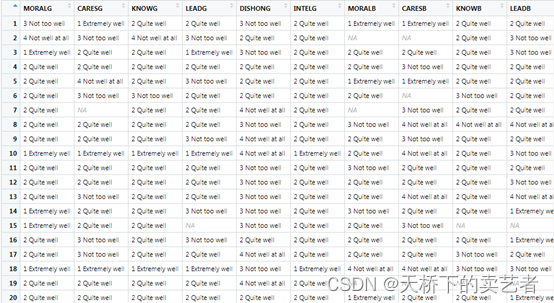poLCA包进行分析变量的formula（公司）基本格式为：

``````f <- cbind(Y1,Y2,Y3)~1
``````

``````f <- cbind(Y1,Y2,Y3)~X1+X2*X3
``````

Y1,Y2,Y3需要是等级变量，在本数据中就是这些问卷的条目,如果不指定协变量，就是

``````f <- cbind(MORALG,CARESG,KNOWG,LEADG,DISHONG,INTELG,MORALB,CARESB,KNOWB,LEADB,DISHONB,INTELB)~1
``````

``````poLCA(formula, data, nclass=2, maxiter=1000, graphs=FALSE, tol=1e-10,
na.rm=TRUE, probs.start=NULL, nrep=1, verbose=TRUE, calc.se=TRUE)
``````

data就是你的数据，nclass代表你要分成几个类型，maxiter表示算法最大迭代次数，默认1000次，graphs等于T的话会生成图形，tol：一个容差值用于判断何时达到收敛，一般默认值就可以了。nrep表示对模型估计的次数，nrep > 1可以自动搜索对数似然函数的全局最大值而不是局部最大值。poLCA只返回产生最大对数似然函数的模型对应的参数估计。

``````nes1 <- poLCA(f,election,nclass=1)  # log-likelihood: -18647.31
``````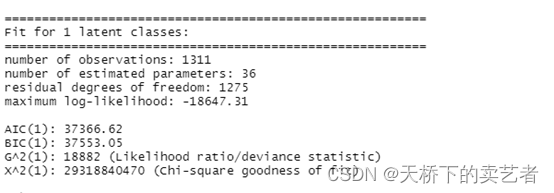``````nes2 <- poLCA(f,election,nclass=2)  # log-likelihood: -17344.92
``````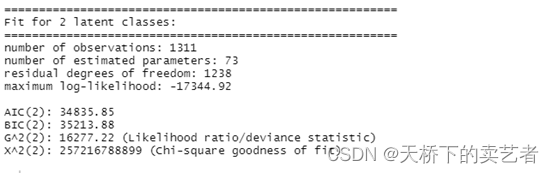``````nes3 <- poLCA(f,election,nclass=3)  # log-likelihood: -16714.66
``````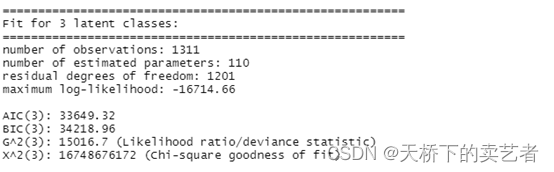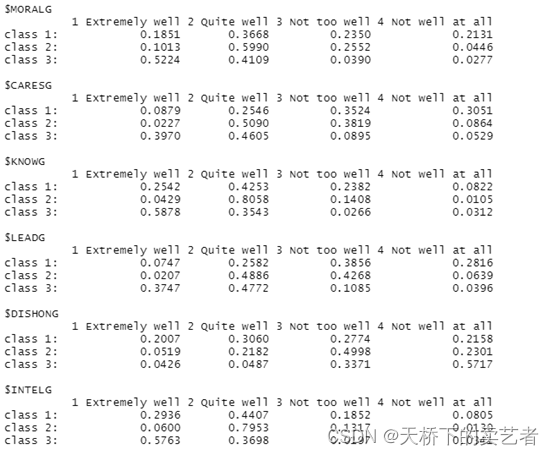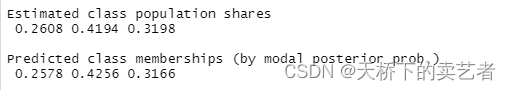``````nes3 <- poLCA(f,election,nclass=3,graphs=T)  # log-likelihood: -16714.66
``````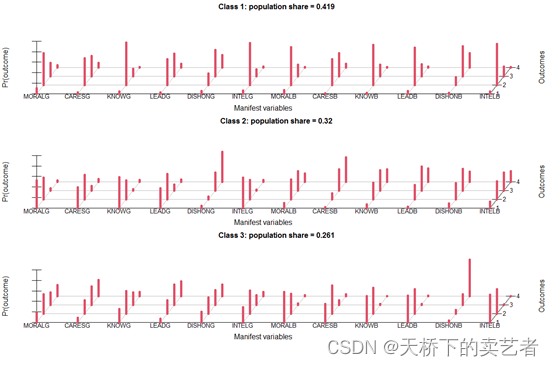``````library(reshape2)
library(ggplot2)dat1<- melt(nes3\$probs, level = 3)ggplot(dat1,aes(x = L3, y = value, fill = Var2)) +geom_bar(stat = 'identity', position = 'stack', width = 0.5) +facet_grid(Var1~.) +scale_fill_brewer(type = 'seq', palette = 'Greys') +theme_bw() +labs(x = '', fill = 'probabilities') +guides(fill = guide_legend(reverse = TRUE))
``````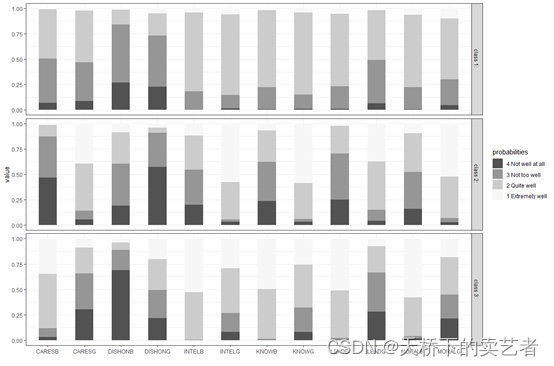``````stat<-NULL
for(i in 2:10){out<- poLCA(f,election,nclass=i,graphs=F) likelihood<-out[["aic"]]stat<-append(stat,likelihood)
}
stat
min(stat)
``````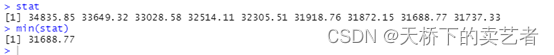``````f.party <- cbind(MORALG,CARESG,KNOWG,LEADG,DISHONG,INTELG,MORALB,CARESB,KNOWB,LEADB,DISHONB,INTELB)~PARTY
nes2a <- poLCA(f2a,election,nclass=3,nrep=5)    # log-likelihood: -16222.32 graphs
``````

``````probs.start <- poLCA.reorder(nes.party\$probs.start,order(nes.party\$P,decreasing=T))
nes.party <- poLCA(f.party,election,nclass=3,probs.start=probs.start)
``````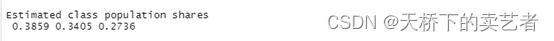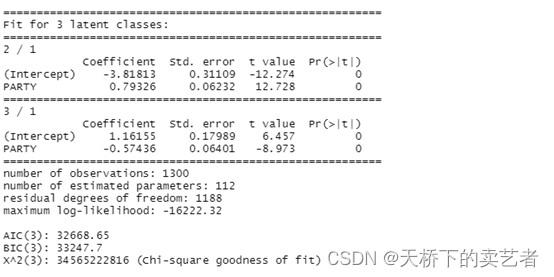R的操作为

``````pidmat <- cbind(1,c(1:7))
exb <- exp(pidmat %*% nes2a\$coeff)
``````

``````matplot(c(1:7),(cbind(1,exb)/(1+rowSums(exb))),ylim=c(0,1),type="l",main="Party ID as a predictor of candidate affinity class",xlab="Party ID: strong Democratic (1) to strong Republican (7)",ylab="Probability of latent class membership",lwd=2,col=1)
text(5.9,0.35,"Other")
text(5.4,0.7,"Bush affinity")
text(1.8,0.6,"Gore affinity")
``````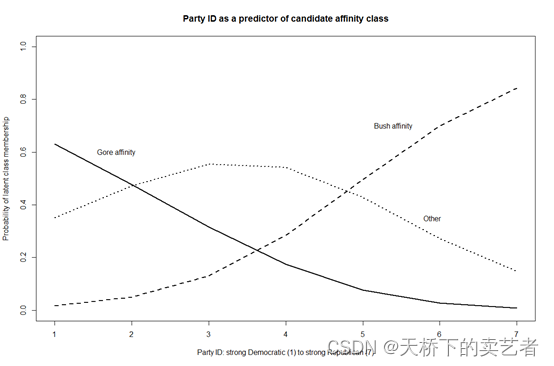``````f.3cov <- cbind(MORALG,CARESG,KNOWG,LEADG,DISHONG,INTELG,MORALB,CARESB,KNOWB,LEADB,DISHONB,INTELB)~PARTY*AGE
nes.3cov <- poLCA(f.3cov,election,nclass=3,verbose=F)
``````

``````probs.start <- poLCA.reorder(nes.3cov\$probs.start,order(nes.3cov\$P,decreasing=T))
nes.3cov <- poLCA(f.3cov,election,nclass=3,probs.start=probs.start)
``````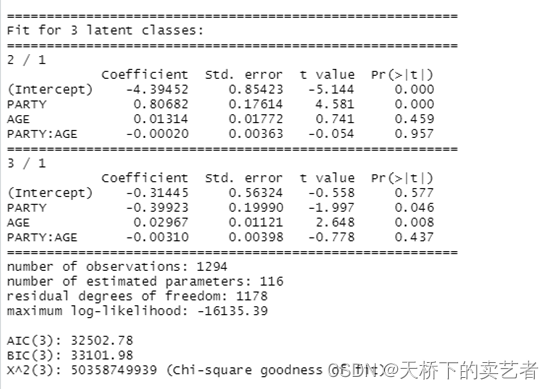``````strdems <- cbind(1,1,c(18:80),(c(18:80)*1))
exb.strdems <- exp(strdems %*% nes.3cov\$coeff)
matplot(c(18:80),(cbind(1,exb.strdems)/(1+rowSums(exb.strdems))),main="Age and candidate affinity for strong Democrats",xlab="Age",ylab="Probability of latent class membership",ylim=c(0,1),type="l",col=1,lwd=3)
text(50,0.6,"Gore affinity")
text(50,0.39,"Other")
text(50,0.1,"Bush affinity")
``````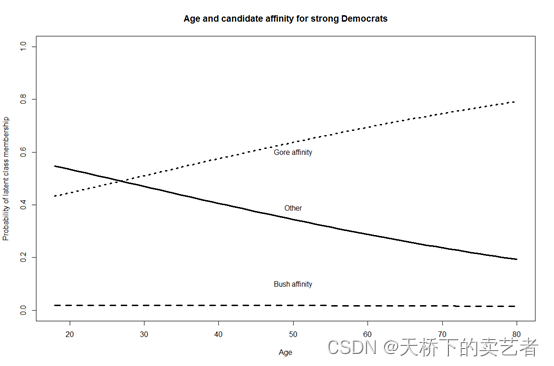``````strreps <- cbind(1,7,c(18:80),(c(18:80)*7))
exb.strreps <- exp(strreps %*% nes.3cov\$coeff)
matplot(c(18:80),(cbind(1,exb.strreps)/(1+rowSums(exb.strreps))),main="Age and candidate affinity for strong Republicans",xlab="Age",ylab="Probability of latent class membership",ylim=c(0,1),type="l",col=1,lwd=3)
text(50,0.8,"Gore affinity")
text(50,0.2,"Other")
text(50,0.05,"Bush affinity")
``````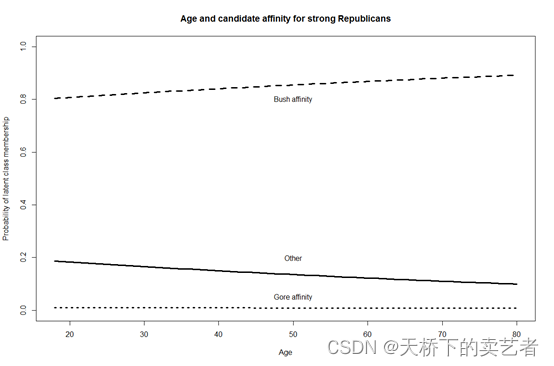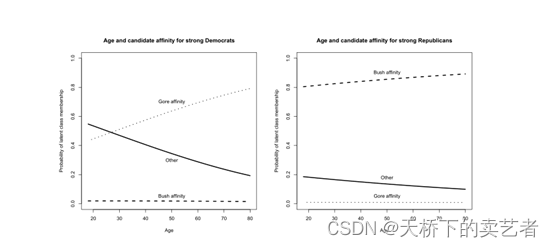1. poLCA包说明
2. 张洁婷, 焦璨, 张敏强. 潜在类别分析技术在心理学研究中的应用[J]. 心理科学进展, 2010(12):8.
3. 房立艳, 张大均, 武丽丽,等. 中学生心理素质的类别特征:基于个体中心的潜在类别分析[J]. 心理与行为研究, 2017, 15(1):6.
4. Mirza S S , Wolters F J , Swanson S A , et al. 10-year trajectories of depressive symptoms and risk of dementia: a population-based study[J]. Lancet Psychiatry, 2016:628-635.
5. https://mp.weixin.qq.com/s/Bvob1r2g6vr7gcavMOydAA

#### 相关文章

linux系统Padavan中U盘硬盘格式化为ext4 fdisk -lumount /dev/sdb1mkfs.ext4 /dev/sdb1如下图 参考资料 http://www.chenyajun.net/?id41

### 【图解HTTP】确保WEB安全的HTTPS

【HTTP的缺点】 【通信使用明文&#xff0c;内容可能会被窃听】 HTTP报文使用明文方式发送 【TCP/IP是可能被窃听的网络】 按TCP/IP协议族的工作机制&#xff0c;通信内容在所有的通信线路上都有可能遭到窥视。 即使经过加密处理的通信&#xff0c;也会被窥视到通信内容&a…

### Linux实操篇-网络配置

1.Linux网络配置原理图&#xff08;含虚拟机&#xff09; 目前我们的网络配置采用的是NAT。 2.查看网络ip和网关 查看虚拟网络编辑器 修改IP地址&#xff08;修改虚拟网络的ip&#xff09; 修改“虚拟网络编辑器”的子网IP 查看网关 &#xff08;网关通常为当前网段的端口&…